﻿ Functions in ReportWorkshop

# Template syntax | Aggregate functions

See the topic about fields in templates for a general description of the syntax.

See the topic about cross-tab reports.

This type of field allows inserting the result of an aggregate function in cross-tab tables.

Aggregate functions are not available for non-cross-tab tables. If you want to add summary row(s) or column(s) for non-cross-tab tables, you can:

calculate them using SQL functions, if you use SQL data queries, or

use OnProcessRecord event to calculate the function in a variable.

## Syntax

<aggregate function> ::= =<function name>(["]<param>["])[ <result type>][ "<format>"]

<function name> ::= min | max | sum | average | count | var.p | var.s | stddev.p | stddev.s | <custom function name>

<param> ::= <field name>

<result type> – see function results types sections below.

<format> – see format strings sections below.

<custom function name> – see "additional functions" below.

This type of field allows inserting the result of an aggregate function in cross-tab tables. It is not valid in all other places.

The argument of this function may be one of numeric value fields (see the topic about cross-tab reports).

In other words, <param> is a name of one of data fields in the result of application of one of Table.RowGenerationRules[].DataQuery, providing that it:

is not listed in Table.CrossTabulation.Levels[].FieldName

is not listed in Table.RowGenerationRules[].KeyFieldNames

have the type either rvrftInteger or rvrftFloat.

A set of values of this parameter (used to apply the function) depends on the place of insertion of this field code:

outside report tables, in a non-cross-tab report tables: not valid (no values)

in rows of a cross-tab header or above: not valid (no values)

in the cells located in the intersection of rows corresponding to RowGenerationRules[] and:

o... data columns of the cross-tab headers: not valid (no values)

o... summary columns of the cross-tab header: values corresponding to this summary columns and this row

o... all other columns: all values corresponding to this row

in the cells located in the intersection of all other rows and

o... data columns of the cross-tab headers: all values of this column

o... summary columns of the cross-tab header: all values corresponding to this summary columns and all rows

o... all other columns: all values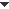Example

## Aggregate functions list

 Meaning Result type Minimal necessary count of input values min returns the smallest value type of the parameter 1 max returns the largest value 1 sum calculates the sum of values 0 count returns the count of values int 0 average returns the average value (arithmetic mean)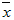float 1 var.p calculates variance (based on the entire population)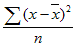1 var.s estimates variance based on a sample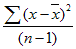2 stddev.p calculates standard deviation (based on the entire population)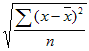1 stddev.s estimates standard deviation based on a sample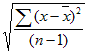2

If the aggregate function cannot be calculated (because it is inserted in a wrong context, or because of not enough input values), a report generator inserts Texts.InvalidFunctionResult in place of the function field. If the aggregate function is used in an expression, and it cannot be calculated, the generator reports an error.

In addition to the functions listed above, programmers can implement their own functions.

As an example, ReportWorkshop includes TRVReportMedianCalculator unit implementing median.

 Meaning Result type Minimal necessary count of input values median calculates the median float 1

## Aggregate functions results types

As you can see from the table above, an aggregate function may return either integer or floating point value.

You can override the result type by specifying <field type>, read the topic about specifying data field types.

## Using aggregate functions in fields of other types

Aggregate functions may be used not only in a special field type; they can also be used in expressions (in fields of expression type, and in \$IF commands).

In expressions, parameters must be enclosed in double quotes, for example: {\$IF Sum("Sales")>0}, or {=Max("Date")-Min("Date")}.

When used separately, double quotes are optional; you can write {Sum(Sales)} or {Sum("Sales")}.